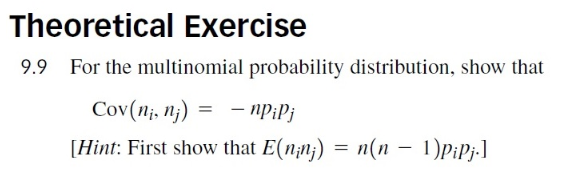Home / Expert Answers / Statistics and Probability / need-help-doing-this-proof-on-r-markdown-9-9-for-the-multinomial-probability-distribution-sh-pa331

# (Solved): Need help doing this proof on R-markdown $$9.9$$ For the multinomial probability distribution, sh ...

Need help doing this proof on R-markdown$$9.9$$ For the multinomial probability distribution, show that $\operatorname{Cov}\left(n_{i}, n_{j}\right)=-n p_{i} p_{j}$ [Hint: First show that $$\left.E\left(n_{i} n_{j}\right)=n(n-1) p_{i} p_{j} .\right]$$

We have an Answer from Expert